or# RD Sharma Class 12 and Chapter Tangents And Normals Maths Solutions

## Maths Class 12 and Chapter Tangents And Normals RD Sharma SolutionsFilter
Filters :
CLASSES
•
•
•
•
•
•
•
CHAPTERS
•
• 24 More

### RD SHARMA Class 12 | TANGENTS AND NORMALS

Find the condition for the following set of curves to intersect orthogonally: (x^2)/(a^2)-(y^2)/(b^2)=1 and x y=c^2 (x^2)/(a^2)+(y^2)/(b^2)=1 and (x^2)/(A^2)-(y^2)/(B^2)=1.### RD SHARMA Class 12 | TANGENTS AND NORMALS

Find the angle of intersection of the following curves : y^2=xa n dx^2=y y=x^2a n dx^2+y^2=20 2y^2=x^3a n dy^2=32 x x^2+y^2-4x-1=0a n dx^2+y^2-2y-9=0 (x^2)/(a^2)+(y^2)/(b^2)=1a n dx^2+y^2=a b x^2+4y^2=8a n dx^2-2y^2=2 x^2=27ya n dy^2=8x x^2+y^2=2xa n dy^2=x y=4-x^2a n dy=x^2A lot of our career based on the selections we made in our school time. And, nothing matters more than the books we study from. Where the books are an integral part of our school life, studying from the right set of books on the other side helps us to excel more in our respective classes.

It is well-known that NCERT books are what most of the students carry throughout their school life if they're a part of CBSE board. But the same books are seemed to be efficient until class 7th due to the limited information they have.

Believe it or not, the books comprise of basic theory, general problems and selective formulas, which altogether make it quite hard for students to learn beyond the syllabus. It is also said by experts that students who only study from NCERT are having less clear basic than those who constantly practice through R.D. Sharma help guides.

You must refer to R.D. Sharma as one of the thickest books that are too heavy to carry and too massive to study. But whatever your excuses are, these help guides are the one-best solution to strengthen your base and brush up your concepts. If you want to be a wiser mind at maths, then get your help guide today and start practicing math numerical from it.

## TANGENTS AND NORMALS

In the previous lesson, you have gone through about the fact that the slope of a line is the tangent of the angle which this line makes with the positive direction of the x-axis. It is denoted by the letter 'm'. If θ is the angle which a line is in direction of the x-axis, then m is given by tan θ. You have also learned that the slope m of a line, passing through two points X1, Y1, and X2, Y2 is given by m= (Y2-Y1)/(X2-X1).

In this lesson, we shall find the equations of tangents and normal to different curves, using the knowledge of differential calculus. In the previous chapter, we have already learned about Rolle's Theorem and Mean Value Theorems and their applications.

## TANGENTS AND NORMALS

This explains, how differentiation can be used to calculate the equations of the tangent and normal to a curve. The tangent is a straight line which touches the curve at a specified point. The normal is a straight line which is right-angle to the tangent.

To calculate the equations of these lines you can use the equation of a straight line passing through the point (x1, y1) and having gradient m is given byy y1 = m

x x1

We can also use the fact with gradients m1 and m2 respectively are perpendicular, then m1m2 = −1.

## 1. Introduction

Suppose, a function f(x) shown in Figure 1. When we calculate the derivative, (f’) of the function at a point x = a, we are finding the gradient of the tangent to the graph of that function at that point. The given graph (Figure 1) shows that the tangent drawn at the point x = a. So, the gradient of this tangent is f(a).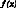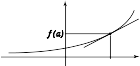Figure 1. The tangent is drawn at point x = a and gradient f(a).

We will use this information to calculate the equation of the tangent to a curve at a point, and then the equation of the normal to a curve at a point.

### Key Point

f(a) is the gradient of the tangent at x = a.

## 2. CALCULATING THE EQUATION OF A TANGENT

Example

Find the equation of the tangent to

f(x) = x3 3x2 + x 1

at the point where x = 3. When x = 3 we note that

f (3) = 33 3.32 + 3 1 = 27 27 + 3 1 = 2

So, the point of intersection is at point (3, 2).

To find the gradient, we need to differentiate f(x):

f(x) = 3x2 - 6x + 1

Now, we will calculate the gradient of the curve at the point where x = 3.

f(3) = 3.32 6.3 + 1 = 27 18 + 1 = 10

So, the coordinates of the required point are (3, 2), and the gradient of the tangent at that point is equal to 10.

Now, we will calculate the equation of the tangent at this point on the curve. The tangent must pass through the point and it must have gradient equal to 10. The tangent is a straight line and so we can equation of a straight line that passes through a point (x1, y1) and has a gradient

m is given by the formulay y1 = m

x x1

Substituting the given values

(y-2)/(x-3) =10

and rearranging

(y-2) =10(x-3)

y-2=10x-30

y=10x-30

this is the equation of the tangent to the curve at (3,2).

### Key Point

The equation of a straight line that passes through a point (x1, y1) and has slope m is given by

(y-y1)/(x-x1)=m

## The equation of a normal to a curve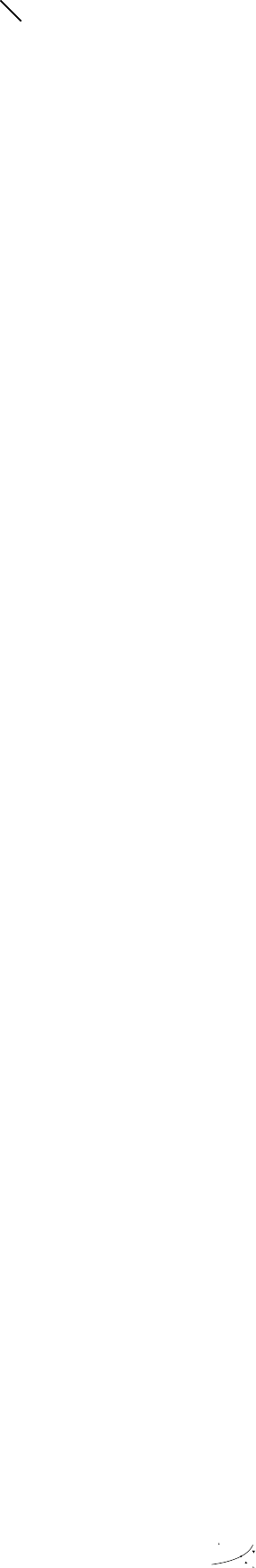In mathematics, the word ‘normal’ has a very precise meaning. It means ‘perpendicular’ or ‘at right angles’.

Tangent normal

If we get a curve which is shown in Figure 2, we can choose a point and draw in the tangent to the curve at that point. The normal is then at right angles to the curve so it is also at right angles to the tangent.

We now find the equation of the normal to a curve. There is another thing that we need in order to do this. If two lines, having gradients m1 and m2 respectively, are at right angles to each other than the product of their gradients, m1m2, must equal −1.

### Key Point

If two lines, having gradients m1 and m2 are at right angles then m1m2 = −1

## Rolle’s Theorem

Suppose f(x)f(x) is a function that satisfies all the following.

1. f(x)f(x) is continuous on the closed interval [a,b].

2. f(x)f(x) is differentiable on the open interval (a,b).

3. f(a)=f(b)f(a)=f(b)

Then there is a number c such that a<c<b and f(c)=0. Or, in other words f(x) has a critical point in (a,b).

### PROOF

For a real h such that c + h is in [a, b], the value f (c + h) is smaller or equal to f (c) because f attains its maximum at c. Therefore, for every h > 0,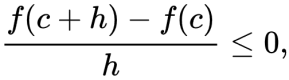Hence,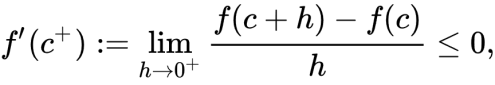Where the limit exists by assumption, it may be minus infinity.

f ′ ( c + ) := lim h → 0 + f ( c + h ) − f ( c ) h ≤ 0 , {\displaystyle f'(c^{+}):=\lim _{h\to 0^{+}}{\frac {f(c+h)-f(c)}{h}}\leq 0,} Similarly, for every h < 0, the inequality changes because the denominator is negative here, so we get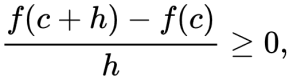f ( c + h ) − f ( c ) h ≥ 0 , {\displaystyle {\frac {f(c+h)-f(c)}{h}}\geq 0,}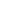Hence,

f ′ ( c − ) := lim h → 0 − f ( c + h ) − f ( c ) h ≥ 0 , {\displaystyle f'(c^{-}):=\lim _{h\to 0^{-}}{\frac {f(c+h)-f(c)}{h}}\geq 0,}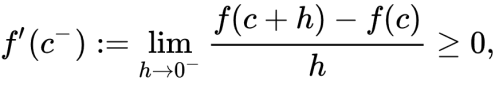Where the limit might be plus infinity.

Finally, when the above right- and left-hand limits agree (when f is differentiable), then the derivative of f at c must be zero.

## SIGNIFICANCE OF RD SHARMA CLASS 12TH MATH

1. RD Sharma books are conspicuously known for the efficient topic smart course and various amounts of preparing to tend to that are slippery in some other practice book.

2. Normally the finding applicants like to practice through this book because it has a ton of request to grasp that empower them to show signs of improvement for last CBSE similarly as other-centered tests.

3. When it comes to science, favorable remedies and practice is a must. Notwithstanding how insightful you are or, how incredible you are with estimations without clarifying different sorts of request, all your undertakings are waste. Thusly, it's more brilliant to go with RD Sharma help guides which have distinctive edge numerical giving you an idea of certified math’s and its complexities.

4. RD Sharma twelfth class math’s books have various preparing questions and exercises to clarify.

5. Conceptualized and accumulated by guidance zone masters, these books are composed by the present test plans. The new form of the book contains all the continuous changes and are twofold checked by experienced educators.

6. As the book involves both, comprehended and unsolved request, the candidates can find the game plans of such practice rehearses online as well. Basically, RD Sharma books are amassed agreeably and upheld with online courses of action.